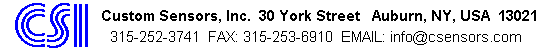# CCD Barcode Scanner, Barcode Reader,Converter

 Barcode Scanners Fixed Beam Scanners Decoders USB Products Converter & Extender Support Return Policy Site map Home Request More Info
How to Calculate a Modulo 10 Check Character.

Modulo 10 check characters are used with various barcode symbologies. They are always used with UPC-A and EAN-13 barcodes. We get quite a number of inquiries as to how to calculate this digit. So here is the procedure.

1. For this process the right most digit is the first digit in the code. (The 11th digit from the left for UPC, the 12th digit from the left for EAN).
2. From right to left add all the odd digits (first, third, etc.) and multiply the total by 3.
3. To this total add the total of the even digits.
4. Subtract the resulting total from the next highest multiple of ten. (If the total was 74, subtract it from 80.)
5. The resulting character is the check digit.

Try this on a few UPC or EAN symbols that have the check digit already printed with the symbol, just to make sure you've got the process correct. If you've got a code with the digit already printed, make sure not to include it in your calculations. Remember, with UPC there should be eleven digits involved in the calculation. Twelve with EAN.